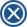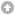Energia Alternativa Laboratory › Motori e Generatori › Turbine › Rigid Wind Turbine with Harmonic Motions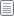Pages: 1 Send TopicPrint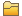Rigid Wind Turbine with Harmonic Motions (Read 732 times)
 victormartin New Member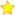OfflineNew EALab Member Posts: 4Rigid Wind Turbine with Harmonic Motions 29.06.18 at 11:01:42 Print Post Hello,I am modeling a rigid offshore floating wind turbine, and like to find AeroDynamic forces on the rotor while the rigid body has harmonic motions in the surge direction in the form of a*cos(w*t) , where "a" is the amplitude of motion, and "w" is the radial frequency of the motion. To model a rigid turbine, in the primary.FST file, in the part "FEATURE FLAGS" I deactivated all the degrees of freedom except CompAero. To give harmonic oscillations to the structure in the surge direction, in the file FAST.f90 in the subroutine solver, I changed the value of Q(displacement), QD(velocity),QD2(acceleration) ,QT,QDT,QD2T for the first degree of freedom (surge DOF) to a harmonic function. In details:The subroutine solver starts with the 2 lines: "QT = Q (:,IC(1))" " QDT = QD(:,IC(1))" before these 2 lines I added the lines :QT (1) =amp1*cos(w*(step+1)*DT)QDT(1) = -amp1*w*sin(w*(step+1)*DT), where amp1 is the amplitude of motion in surge, and w is the radial frequency.The next lines in the subroutine solver are related to RK4 time integration method. In each substep, I used the same expressions as above to redefine the displacement and velocity in the surge direction. In the last substep of RK4,where the final values of Q,QD are calculated I added the lines:Q (1,IC(NMX)) =amp1*cos(w*(step+1)*DT)QD(1,IC(NMX)) = -amp1*w*sin(w*(step+1)*DT)The next lines in the subroutine solver are related to Adams-Bashforth predictor, in this part I added 2 lines the same as the above lines. After the predictor, there is the line "QD2(:,IC(NMX)) = QD2T" before this line I added:QD2T(1)=-amp1*w**2*cos(w*(step+1)*DT)In the Corrector (Adams-Moulton) part, I added the lines:Q (1,IC(NMX)) =amp1*cos(w*(step+1)*DT)QD(1,IC(NMX)) = -amp1*w*sin(w*(step+1)*DT)After the corrector part there is the line: "QD2(:,IC(NMX)) = QD2T" before this line I added:QD2T(1)=-amp1*w**2*cos(w*(step+1)*DT)I really appreciate it if anyone could tell me whether these changes in the source code are enough to model a rigid wind turbine with harmonic oscillations in the surge direction or not.Please helpI didn't find the right solution from the internet.References:https://wind.nrel.gov/forum/wind/viewtopic.php?t=831Animated Explainer Video Production StudioThank you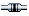IP LoggedPages: 1 Send TopicPrint

 « Board Index ‹ Board